# # R-IoT compatibility with W3C `devicemotion` specification

The aim of this document is to describe a convention to normalize data provided by the R-IoT motion sensor to match the W3C devicemotion API (opens new window).

## # Goal

### # Smartphone and R-IoT axes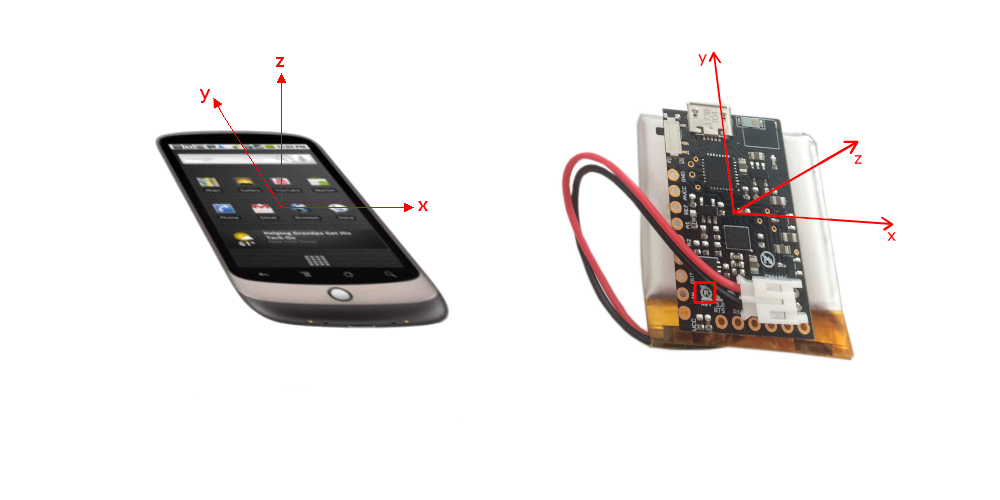### # Units

Smartphone R-IoT
Accelerometer m/s2 g
Gyroscope °/s °/ms

### # Mapping function

To follow the `devicemotion` specification, the R-IoT's original acceleration and gyroscope data must be changed as follows:

``````const tmp_x = x;

// Acceleration : from g to m/s2 --> multiply by 9.81
x = 9.81 * y;
y = -9.81 * tmp_x;
z = 9.81 * z;

const tmp_alpha = alpha;

// Rotation : from °/ms to °/s --> multiply by 1000
alpha = 1000 * gamma;
beta = 1000 * beta;
gamma = -1000 * tmp_alpha;

``````

## # Smartphone's motion formats

Smartphone's precise specifications can be found here : https://www.w3.org/TR/orientation-event/#introduction (opens new window).

From the deviceMotionEvent , we can get the accelerationIncludingGravity and rotationRate. The axes are set such as, with the phone held on the vertical, screen side up, x and y axis are both in the same plane, the first going towards the right, and the latter going up, and the z axis perpendicular to the screen.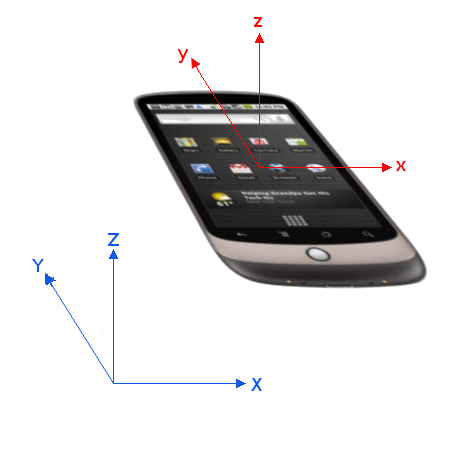The accelerationIncludingGravity return 3 floats, one for each axis, and is expressed in m/s2. For example, if the phone is sitting flat on a table, screen side up, the acceleration would be [0, 0, 9.81].

As for the rotationRate, it returns 3 floats for each angular velocity, in °/sec. The variable alpha represents the rotation angle around the the z axis,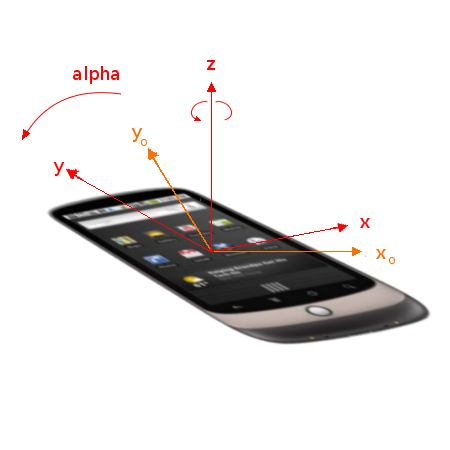beta around the x axis,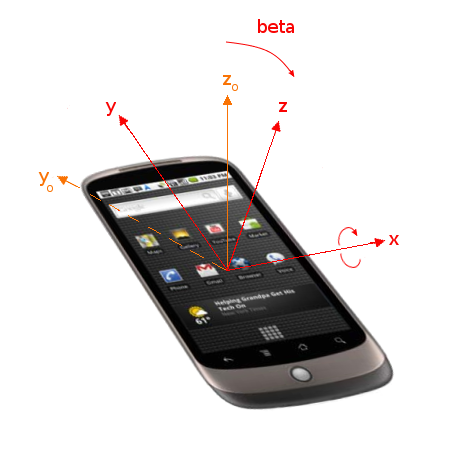and gamma around the y axis.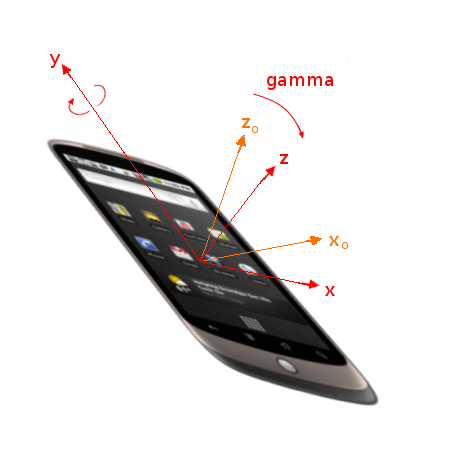## # R-IoT

See the R-IoT manual : https://bitalino.com/docs/MANUAL_RIOT_V1.2.pdf (opens new window).

By default, the acceleration and rotation data from the R-IoT ranges between -1 and 1, expressed in m/s2 and °/ms, respectively.

As for the axes, by default they are as follows :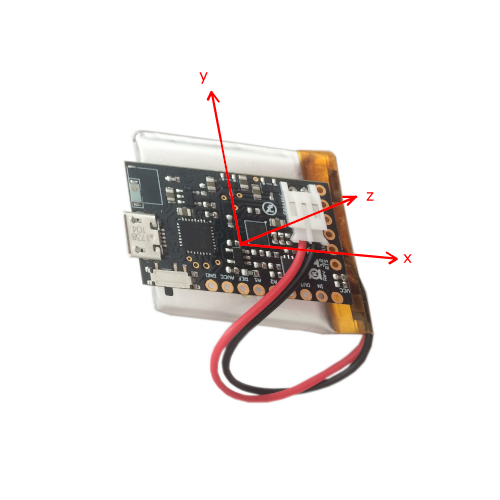However, we decided that we would change the axes so that the R-IoT indication on the sensor is legible. The axes thus need to be changed from this :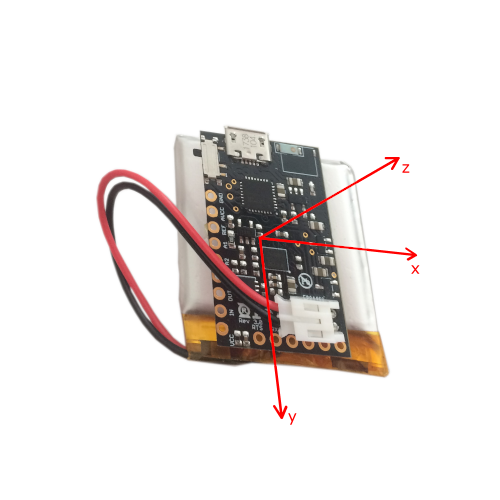to this :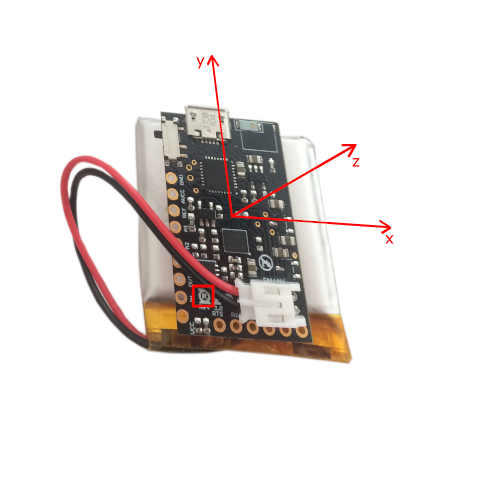As for the angles, gamma is the angle around the z axis, beta around the x axis, and alpha around the y axis.

{Sound Music Movement} Interaction Team - STMS-LAB